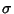## Sample Variance

To estimate the population Variance from a sample ofelements with a priori unknown Mean (i.e., the Mean is estimated from the sample itself), we need an unbiased Estimator for. This is the k-Statistic, where(1)

andis the sample variance(2)

Note that some authors prefer the definition(3)

since this makes the sample variance an Unbiased Estimator for the population variance.